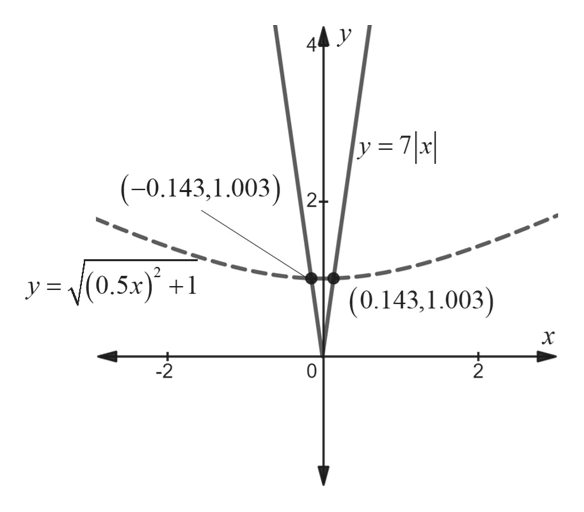Find the solutions of the inequality by drawing appropriate graphs. State the answer rounded to two decimals. (Enter your answer using interval notation.) ((0.5x)^2 + 1) ^(1/2) ≤ 7|x|

Question

Find the solutions of the inequality by drawing appropriate graphs. State the answer rounded to two decimals. (Enter your answer using interval notation.)

((0.5x)^2 + 1) ^(1/2) ≤ 7|x|
Step 1

Sketch the graphs y = ((0.5x)^2+1)^1/2 and y =...help_outlineImage Transcriptionclose|y = 7 \a| (-0.143,1.003)2! (0.5x + y= (0.143,1.003) 10 2 fullscreen

Want to see the full answer?

See Solution

Want to see this answer and more?

Our solutions are written by experts, many with advanced degrees, and available 24/7

See Solution
Tagged in

Equations and In-equations